Courses

# Test: Data Handling - 2

## 10 Questions MCQ Test Mathematics for Class 5 (V) - CBSE and NCERT Curriculum | Test: Data Handling - 2

Description
This mock test of Test: Data Handling - 2 for Class 5 helps you for every Class 5 entrance exam. This contains 10 Multiple Choice Questions for Class 5 Test: Data Handling - 2 (mcq) to study with solutions a complete question bank. The solved questions answers in this Test: Data Handling - 2 quiz give you a good mix of easy questions and tough questions. Class 5 students definitely take this Test: Data Handling - 2 exercise for a better result in the exam. You can find other Test: Data Handling - 2 extra questions, long questions & short questions for Class 5 on EduRev as well by searching above.
QUESTION: 1

Solution:
QUESTION: 2

### Direction: The bar graph shows the mass of 5 children.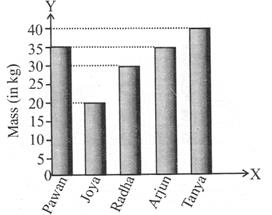Q. What is the difference between the mass of the heaviest child and the lightest child?

Solution:

Mass of the heaviest child = 40 kg Mass of the lightest child = 20 kg Their difference = 20 kg

QUESTION: 3

### Direction: The bar graph shows the mass of 5 children.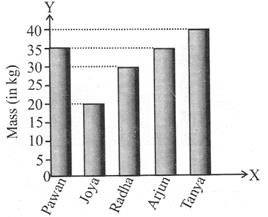Q. Which of the two children have the same mass?

Solution:

From the graph, the two bars corresponding to the mass of Pawan and Arjun are of the same height. So, Pawan and Arjun have the same mass.

QUESTION: 4

Direction: The bar graph shows the mass of 5 children.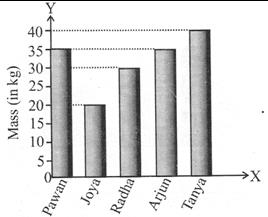Who is 20 kg heavier than Joya?

Solution:

Mass of Joya = 20 kg 20 kg heavier than Joya = 20 kg + 20 kg = 40 kg = Mass of Tanya

QUESTION: 5

Direction: The graph shows how Lalit spends his pocket money each month.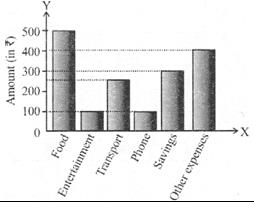Q. The amount spent on food is how many times the amount spent on transport?

Solution:
QUESTION: 6

Direction: The graph shows how Lalit spends his pocket money each month.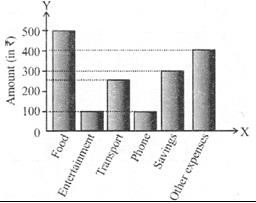Q. What is the fraction of the amount spent on other expenses to the amount spent on food?

Solution:
QUESTION: 7

Direction: The graph shows how Lalit spends his pocket money each month.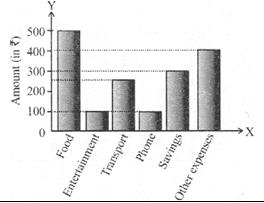How much does Lalit earn per month?

Solution:
QUESTION: 8

The pie-chart shows the modes of transport used by students of a school. XY is a straight line and 0 is the centre of the circle. If 45 students go to school by taxi, how many of them go by scooter?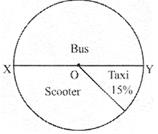Solution:
QUESTION: 9

Direction: The line graph shows the money earned by a fruit seller during a certain week.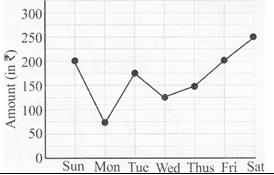On which day did he earn Rs.75 less than the amount earned on Thursday?

Solution:

Amount earned on Thursday = Rs. 150

∴  Rs. 75 less than Rs.150 is Rs. 75 = Amount earned on Monday

QUESTION: 10

Direction: The line graph shows the money earned by a fruit seller during a certain week..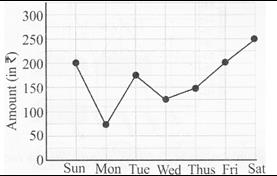What is the average amount earned from Monday to Thursday?

Solution:

Average amount earned from Monday to Thursday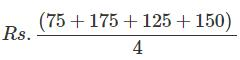= 131.25